# Electrochemistry.pdf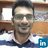Assistant Professor at University of Engineering and Technology Lahore, (RCET) en University of Engineering and Technology Lahore, Gujranwala Campus
27 de May de 2023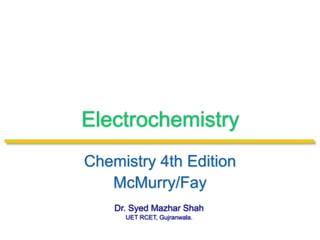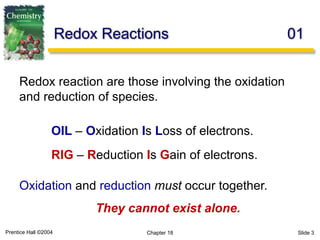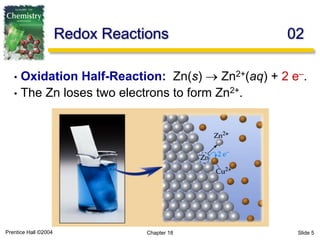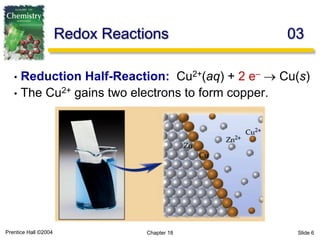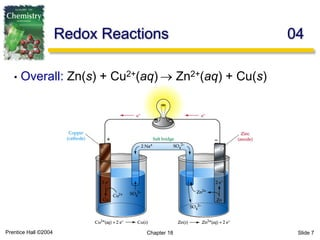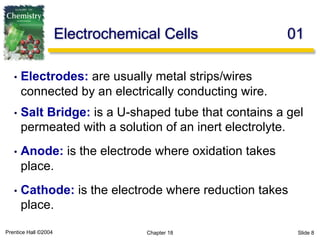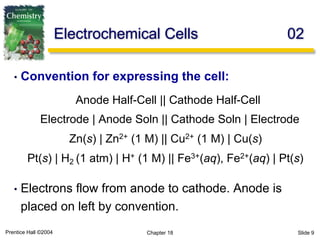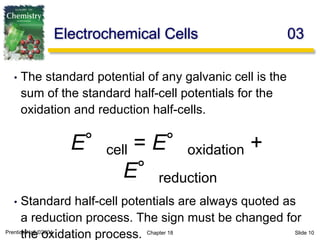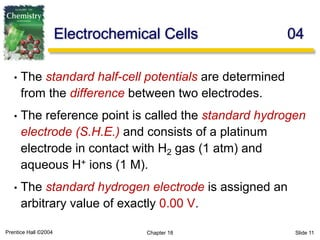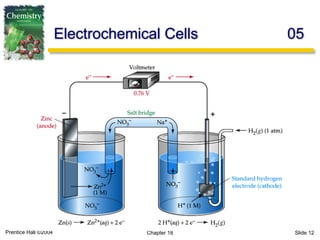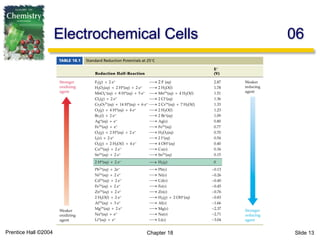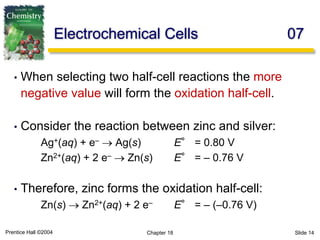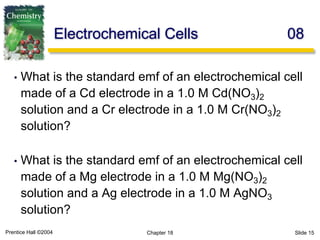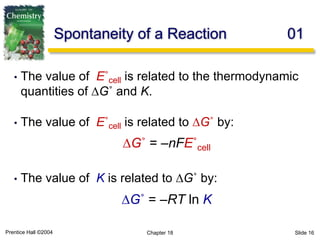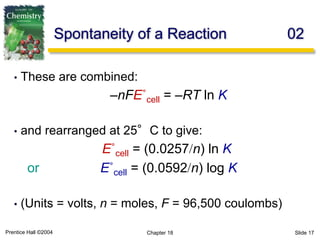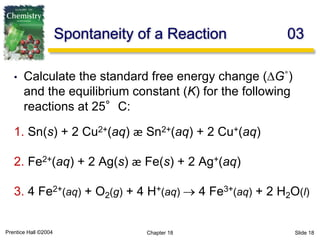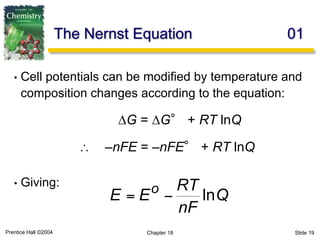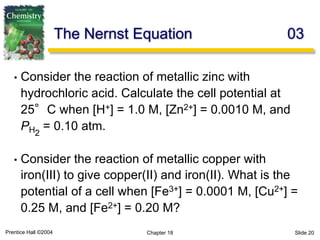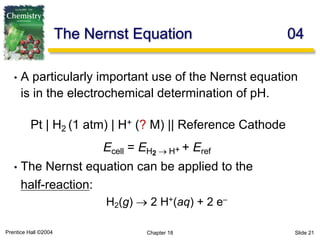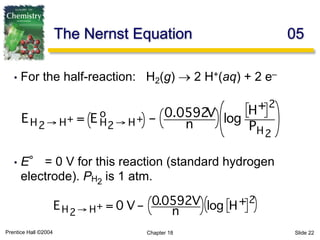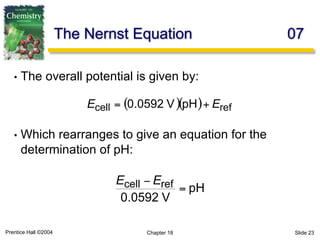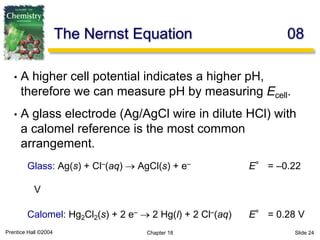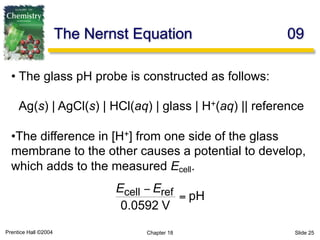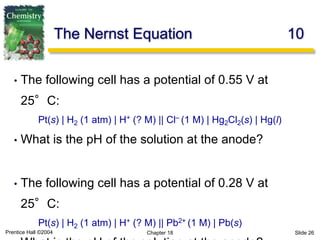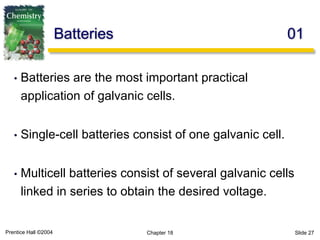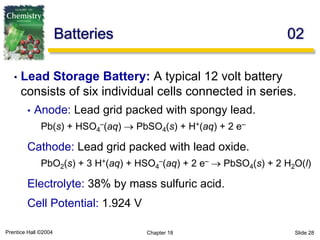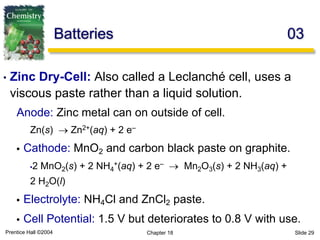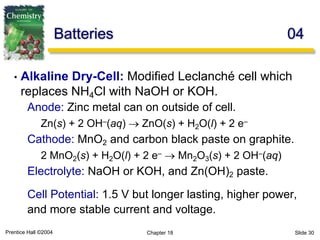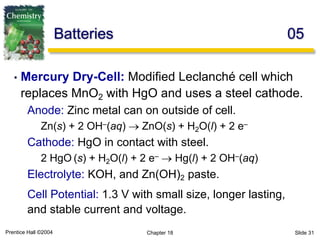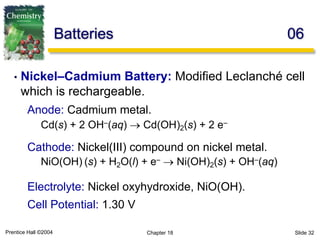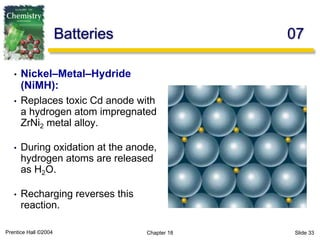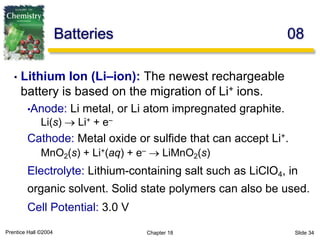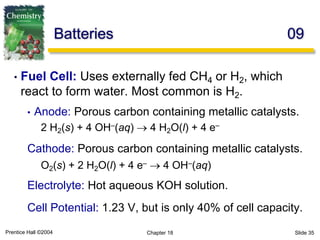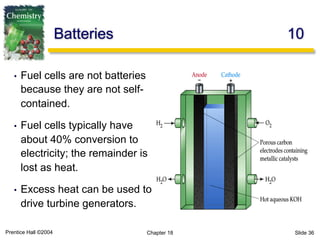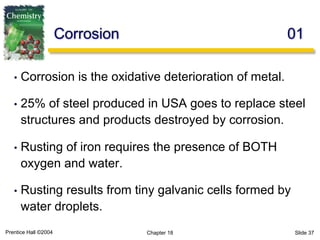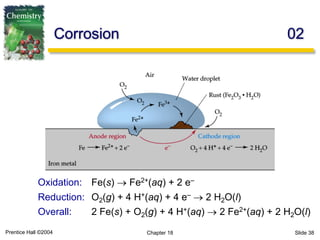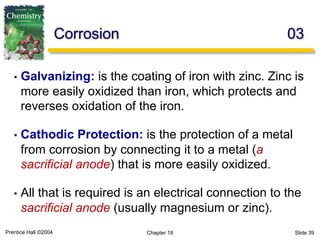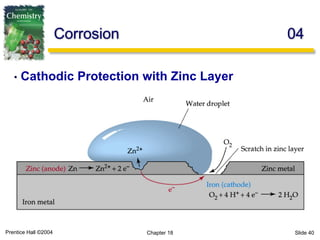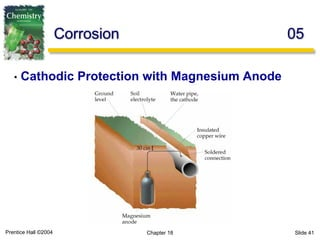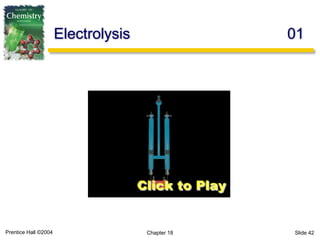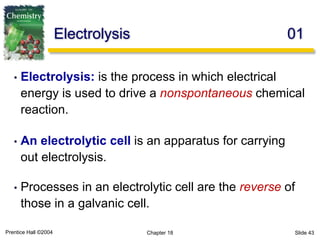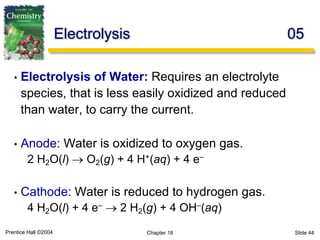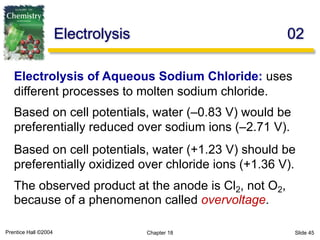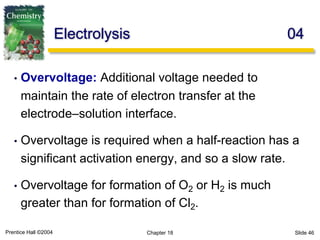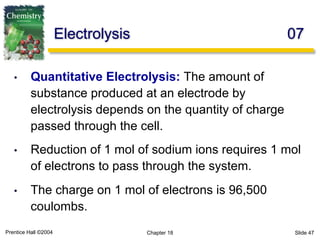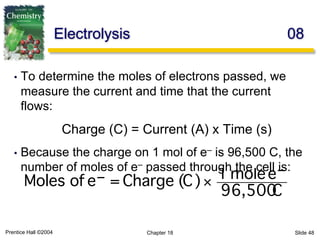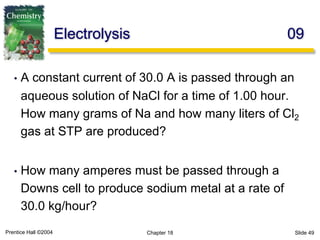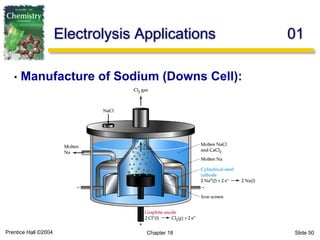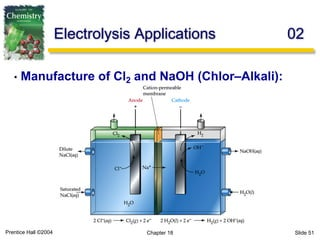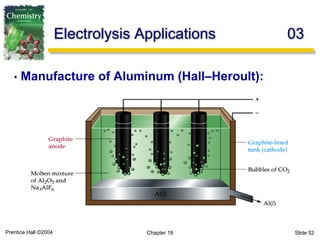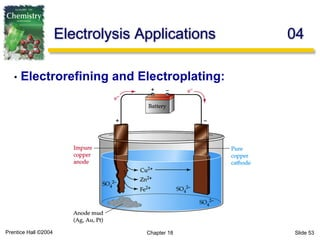1 de 53

### Electrochemistry.pdf

• 1. Dr. Syed Mazhar Shah UET RCET, Gujranwala. Electrochemistry Chemistry 4th Edition McMurry/Fay
• 2. Prentice Hall ©2004 Chapter 18 Slide 2 Redox Reactions 01
• 3. Prentice Hall ©2004 Chapter 18 Slide 3 Redox reaction are those involving the oxidation and reduction of species. OIL – Oxidation Is Loss of electrons. RIG – Reduction Is Gain of electrons. Oxidation and reduction must occur together. They cannot exist alone. Redox Reactions 01
• 4. Prentice Hall ©2004 Chapter 18 Slide 4 Redox Reactions 02
• 5. Prentice Hall ©2004 Chapter 18 Slide 5 • Oxidation Half-Reaction: Zn(s) ® Zn2+(aq) + 2 e–. • The Zn loses two electrons to form Zn2+. Redox Reactions 02
• 6. Prentice Hall ©2004 Chapter 18 Slide 6 Redox Reactions 03 • Reduction Half-Reaction: Cu2+(aq) + 2 e– ® Cu(s) • The Cu2+ gains two electrons to form copper.
• 7. Prentice Hall ©2004 Chapter 18 Slide 7 • Overall: Zn(s) + Cu2+(aq) ® Zn2+(aq) + Cu(s) Redox Reactions 04
• 8. Prentice Hall ©2004 Chapter 18 Slide 8 Electrochemical Cells 01 • Electrodes: are usually metal strips/wires connected by an electrically conducting wire. • Salt Bridge: is a U-shaped tube that contains a gel permeated with a solution of an inert electrolyte. • Anode: is the electrode where oxidation takes place. • Cathode: is the electrode where reduction takes place.
• 9. Prentice Hall ©2004 Chapter 18 Slide 9 Electrochemical Cells 02 • Convention for expressing the cell: Anode Half-Cell || Cathode Half-Cell Electrode | Anode Soln || Cathode Soln | Electrode Zn(s) | Zn2+ (1 M) || Cu2+ (1 M) | Cu(s) Pt(s) | H2 (1 atm) | H+ (1 M) || Fe3+(aq), Fe2+(aq) | Pt(s) • Electrons flow from anode to cathode. Anode is placed on left by convention.
• 10. Prentice Hall ©2004 Chapter 18 Slide 10 Electrochemical Cells 03 • The standard potential of any galvanic cell is the sum of the standard half-cell potentials for the oxidation and reduction half-cells. E°cell = E°oxidation + E°reduction • Standard half-cell potentials are always quoted as a reduction process. The sign must be changed for the oxidation process.
• 11. Prentice Hall ©2004 Chapter 18 Slide 11 Electrochemical Cells 04 • The standard half-cell potentials are determined from the difference between two electrodes. • The reference point is called the standard hydrogen electrode (S.H.E.) and consists of a platinum electrode in contact with H2 gas (1 atm) and aqueous H+ ions (1 M). • The standard hydrogen electrode is assigned an arbitrary value of exactly 0.00 V.
• 12. Prentice Hall ©2004 Chapter 18 Slide 12 Electrochemical Cells 05
• 13. Prentice Hall ©2004 Chapter 18 Slide 13 Electrochemical Cells 06
• 14. Prentice Hall ©2004 Chapter 18 Slide 14 Electrochemical Cells 07 • When selecting two half-cell reactions the more negative value will form the oxidation half-cell. • Consider the reaction between zinc and silver: Ag+(aq) + e– ® Ag(s) E° = 0.80 V Zn2+(aq) + 2 e– ® Zn(s) E° = – 0.76 V • Therefore, zinc forms the oxidation half-cell: Zn(s) ® Zn2+(aq) + 2 e– E° = – (–0.76 V)
• 15. Prentice Hall ©2004 Chapter 18 Slide 15 Electrochemical Cells 08 • What is the standard emf of an electrochemical cell made of a Cd electrode in a 1.0 M Cd(NO3)2 solution and a Cr electrode in a 1.0 M Cr(NO3)2 solution? • What is the standard emf of an electrochemical cell made of a Mg electrode in a 1.0 M Mg(NO3)2 solution and a Ag electrode in a 1.0 M AgNO3 solution?
• 16. Prentice Hall ©2004 Chapter 18 Slide 16 Spontaneity of a Reaction 01 • The value of E˚cell is related to the thermodynamic quantities of ∆G˚ and K. • The value of E˚cell is related to ∆G˚ by: ∆G˚ = –nFE˚cell • The value of K is related to ∆G˚ by: ∆G˚ = –RT ln K
• 17. Prentice Hall ©2004 Chapter 18 Slide 17 Spontaneity of a Reaction 02 • These are combined: –nFE˚cell = –RT ln K • and rearranged at 25°C to give: E˚cell = (0.0257/n) ln K or E˚cell = (0.0592/n) log K • (Units = volts, n = moles, F = 96,500 coulombs)
• 18. Prentice Hall ©2004 Chapter 18 Slide 18 Spontaneity of a Reaction 03 • Calculate the standard free energy change (∆G˚) and the equilibrium constant (K) for the following reactions at 25°C: 1. Sn(s) + 2 Cu2+(aq) æ Sn2+(aq) + 2 Cu+(aq) 2. Fe2+(aq) + 2 Ag(s) æ Fe(s) + 2 Ag+(aq) 3. 4 Fe2+(aq) + O2(g) + 4 H+(aq) ® 4 Fe3+(aq) + 2 H2O(l)
• 19. Prentice Hall ©2004 Chapter 18 Slide 19 • Cell potentials can be modified by temperature and composition changes according to the equation: ∆G = ∆G° + RT lnQ –nFE = –nFE° + RT lnQ • Giving: The Nernst Equation 01 Q nF RT E E o ln − =
• 20. Prentice Hall ©2004 Chapter 18 Slide 20 • Consider the reaction of metallic zinc with hydrochloric acid. Calculate the cell potential at 25°C when [H+] = 1.0 M, [Zn2+] = 0.0010 M, and PH2 = 0.10 atm. • Consider the reaction of metallic copper with iron(III) to give copper(II) and iron(II). What is the potential of a cell when [Fe3+] = 0.0001 M, [Cu2+] = 0.25 M, and [Fe2+] = 0.20 M? The Nernst Equation 03
• 21. Prentice Hall ©2004 Chapter 18 Slide 21 The Nernst Equation 04 • A particularly important use of the Nernst equation is in the electrochemical determination of pH. Pt | H2 (1 atm) | H+ (? M) || Reference Cathode Ecell = EH2 ® H+ + Eref • The Nernst equation can be applied to the half-reaction: H2(g) ® 2 H+(aq) + 2 e–
• 22. Prentice Hall ©2004 Chapter 18 Slide 22 The Nernst Equation 05 • For the half-reaction: H2(g) ® 2 H+(aq) + 2 e– • E° = 0 V for this reaction (standard hydrogen electrode). PH2 is 1 atm. EH2→ H+ = E H2→ H+ o – 0.0592 V n log H+ 2 PH2 EH2→ H+ = 0 V – 0.0592V n log H+ 2
• 23. Prentice Hall ©2004 Chapter 18 Slide 23 The Nernst Equation 07 • The overall potential is given by: • Which rearranges to give an equation for the determination of pH: pH V 0.0592 ref cell = − E E ( )( ) ref cell pH V 0592 . 0 E E + =
• 24. Prentice Hall ©2004 Chapter 18 Slide 24 The Nernst Equation 08 • A higher cell potential indicates a higher pH, therefore we can measure pH by measuring Ecell. • A glass electrode (Ag/AgCl wire in dilute HCl) with a calomel reference is the most common arrangement. Glass: Ag(s) + Cl–(aq) ® AgCl(s) + e– E° = –0.22 V Calomel: Hg2Cl2(s) + 2 e– ® 2 Hg(l) + 2 Cl–(aq) E° = 0.28 V
• 25. Prentice Hall ©2004 Chapter 18 Slide 25 The Nernst Equation 09 • The glass pH probe is constructed as follows: Ag(s) | AgCl(s) | HCl(aq) | glass | H+(aq) || reference •The difference in [H+] from one side of the glass membrane to the other causes a potential to develop, which adds to the measured Ecell. pH V 0.0592 ref cell = − E E
• 26. Prentice Hall ©2004 Chapter 18 Slide 26 The Nernst Equation 10 • The following cell has a potential of 0.55 V at 25°C: Pt(s) | H2 (1 atm) | H+ (? M) || Cl– (1 M) | Hg2Cl2(s) | Hg(l) • What is the pH of the solution at the anode? • The following cell has a potential of 0.28 V at 25°C: Pt(s) | H2 (1 atm) | H+ (? M) || Pb2+ (1 M) | Pb(s)
• 27. Prentice Hall ©2004 Chapter 18 Slide 27 Batteries 01 • Batteries are the most important practical application of galvanic cells. • Single-cell batteries consist of one galvanic cell. • Multicell batteries consist of several galvanic cells linked in series to obtain the desired voltage.
• 28. Prentice Hall ©2004 Chapter 18 Slide 28 Batteries 02 • Lead Storage Battery: A typical 12 volt battery consists of six individual cells connected in series. • Anode: Lead grid packed with spongy lead. Pb(s) + HSO4 –(aq) ® PbSO4(s) + H+(aq) + 2 e– Cathode: Lead grid packed with lead oxide. PbO2(s) + 3 H+(aq) + HSO4 –(aq) + 2 e– ® PbSO4(s) + 2 H2O(l) Electrolyte: 38% by mass sulfuric acid. Cell Potential: 1.924 V
• 29. Prentice Hall ©2004 Chapter 18 Slide 29 Batteries 03 • Zinc Dry-Cell: Also called a Leclanché cell, uses a viscous paste rather than a liquid solution. Anode: Zinc metal can on outside of cell. Zn(s) ® Zn2+(aq) + 2 e– • Cathode: MnO2 and carbon black paste on graphite. •2 MnO2(s) + 2 NH4 +(aq) + 2 e– ® Mn2O3(s) + 2 NH3(aq) + 2 H2O(l) • Electrolyte: NH4Cl and ZnCl2 paste. • Cell Potential: 1.5 V but deteriorates to 0.8 V with use.
• 30. Prentice Hall ©2004 Chapter 18 Slide 30 Batteries 04 • Alkaline Dry-Cell: Modified Leclanché cell which replaces NH4Cl with NaOH or KOH. Anode: Zinc metal can on outside of cell. Zn(s) + 2 OH–(aq) ® ZnO(s) + H2O(l) + 2 e– Cathode: MnO2 and carbon black paste on graphite. 2 MnO2(s) + H2O(l) + 2 e– ® Mn2O3(s) + 2 OH–(aq) Electrolyte: NaOH or KOH, and Zn(OH)2 paste. Cell Potential: 1.5 V but longer lasting, higher power, and more stable current and voltage.
• 31. Prentice Hall ©2004 Chapter 18 Slide 31 Batteries 05 • Mercury Dry-Cell: Modified Leclanché cell which replaces MnO2 with HgO and uses a steel cathode. Anode: Zinc metal can on outside of cell. Zn(s) + 2 OH–(aq) ® ZnO(s) + H2O(l) + 2 e– Cathode: HgO in contact with steel. 2 HgO (s) + H2O(l) + 2 e– ® Hg(l) + 2 OH–(aq) Electrolyte: KOH, and Zn(OH)2 paste. Cell Potential: 1.3 V with small size, longer lasting, and stable current and voltage.
• 32. Prentice Hall ©2004 Chapter 18 Slide 32 Batteries 06 • Nickel–Cadmium Battery: Modified Leclanché cell which is rechargeable. Anode: Cadmium metal. Cd(s) + 2 OH–(aq) ® Cd(OH)2(s) + 2 e– Cathode: Nickel(III) compound on nickel metal. NiO(OH) (s) + H2O(l) + e– ® Ni(OH)2(s) + OH–(aq) Electrolyte: Nickel oxyhydroxide, NiO(OH). Cell Potential: 1.30 V
• 33. Prentice Hall ©2004 Chapter 18 Slide 33 Batteries 07 • Nickel–Metal–Hydride (NiMH): • Replaces toxic Cd anode with a hydrogen atom impregnated ZrNi2 metal alloy. • During oxidation at the anode, hydrogen atoms are released as H2O. • Recharging reverses this reaction.
• 34. Prentice Hall ©2004 Chapter 18 Slide 34 Batteries 08 • Lithium Ion (Li–ion): The newest rechargeable battery is based on the migration of Li+ ions. •Anode: Li metal, or Li atom impregnated graphite. Li(s) ® Li+ + e– Cathode: Metal oxide or sulfide that can accept Li+. MnO2(s) + Li+(aq) + e– ® LiMnO2(s) Electrolyte: Lithium-containing salt such as LiClO4, in organic solvent. Solid state polymers can also be used. Cell Potential: 3.0 V
• 35. Prentice Hall ©2004 Chapter 18 Slide 35 Batteries 09 • Fuel Cell: Uses externally fed CH4 or H2, which react to form water. Most common is H2. • Anode: Porous carbon containing metallic catalysts. 2 H2(s) + 4 OH–(aq) ® 4 H2O(l) + 4 e– Cathode: Porous carbon containing metallic catalysts. O2(s) + 2 H2O(l) + 4 e– ® 4 OH–(aq) Electrolyte: Hot aqueous KOH solution. Cell Potential: 1.23 V, but is only 40% of cell capacity.
• 36. Prentice Hall ©2004 Chapter 18 Slide 36 Batteries 10 • Fuel cells are not batteries because they are not self- contained. • Fuel cells typically have about 40% conversion to electricity; the remainder is lost as heat. • Excess heat can be used to drive turbine generators.
• 37. Prentice Hall ©2004 Chapter 18 Slide 37 Corrosion 01 • Corrosion is the oxidative deterioration of metal. • 25% of steel produced in USA goes to replace steel structures and products destroyed by corrosion. • Rusting of iron requires the presence of BOTH oxygen and water. • Rusting results from tiny galvanic cells formed by water droplets.
• 38. Prentice Hall ©2004 Chapter 18 Slide 38 Corrosion 02 Oxidation: Fe(s) ® Fe2+(aq) + 2 e– Reduction: O2(g) + 4 H+(aq) + 4 e– ® 2 H2O(l) Overall: 2 Fe(s) + O2(g) + 4 H+(aq) ® 2 Fe2+(aq) + 2 H2O(l)
• 39. Prentice Hall ©2004 Chapter 18 Slide 39 Corrosion 03 • Galvanizing: is the coating of iron with zinc. Zinc is more easily oxidized than iron, which protects and reverses oxidation of the iron. • Cathodic Protection: is the protection of a metal from corrosion by connecting it to a metal (a sacrificial anode) that is more easily oxidized. • All that is required is an electrical connection to the sacrificial anode (usually magnesium or zinc).
• 40. Prentice Hall ©2004 Chapter 18 Slide 40 Corrosion 04 • Cathodic Protection with Zinc Layer
• 41. Prentice Hall ©2004 Chapter 18 Slide 41 Corrosion 05 • Cathodic Protection with Magnesium Anode
• 42. Prentice Hall ©2004 Chapter 18 Slide 42 Electrolysis 01
• 43. Prentice Hall ©2004 Chapter 18 Slide 43 • Electrolysis: is the process in which electrical energy is used to drive a nonspontaneous chemical reaction. • An electrolytic cell is an apparatus for carrying out electrolysis. • Processes in an electrolytic cell are the reverse of those in a galvanic cell. Electrolysis 01
• 44. Prentice Hall ©2004 Chapter 18 Slide 44 Electrolysis 05 • Electrolysis of Water: Requires an electrolyte species, that is less easily oxidized and reduced than water, to carry the current. • Anode: Water is oxidized to oxygen gas. 2 H2O(l) ® O2(g) + 4 H+(aq) + 4 e– • Cathode: Water is reduced to hydrogen gas. 4 H2O(l) + 4 e– ® 2 H2(g) + 4 OH–(aq)
• 45. Prentice Hall ©2004 Chapter 18 Slide 45 Electrolysis 02 • Electrolysis of Molten Sodium Chloride: Electrolysis of Aqueous Sodium Chloride: uses different processes to molten sodium chloride. Based on cell potentials, water (–0.83 V) would be preferentially reduced over sodium ions (–2.71 V). Based on cell potentials, water (+1.23 V) should be preferentially oxidized over chloride ions (+1.36 V). The observed product at the anode is Cl2, not O2, because of a phenomenon called overvoltage.
• 46. Prentice Hall ©2004 Chapter 18 Slide 46 Electrolysis 04 • Overvoltage: Additional voltage needed to maintain the rate of electron transfer at the electrode–solution interface. • Overvoltage is required when a half-reaction has a significant activation energy, and so a slow rate. • Overvoltage for formation of O2 or H2 is much greater than for formation of Cl2.
• 47. Prentice Hall ©2004 Chapter 18 Slide 47 Electrolysis 07 • Quantitative Electrolysis: The amount of substance produced at an electrode by electrolysis depends on the quantity of charge passed through the cell. • Reduction of 1 mol of sodium ions requires 1 mol of electrons to pass through the system. • The charge on 1 mol of electrons is 96,500 coulombs.
• 48. Prentice Hall ©2004 Chapter 18 Slide 48 Electrolysis 08 • To determine the moles of electrons passed, we measure the current and time that the current flows: Charge (C) = Current (A) x Time (s) • Because the charge on 1 mol of e– is 96,500 C, the number of moles of e– passed through the cell is: Moles of e– = Charge (C)× 1 molee– 96,500 C
• 49. Prentice Hall ©2004 Chapter 18 Slide 49 Electrolysis 09 • A constant current of 30.0 A is passed through an aqueous solution of NaCl for a time of 1.00 hour. How many grams of Na and how many liters of Cl2 gas at STP are produced? • How many amperes must be passed through a Downs cell to produce sodium metal at a rate of 30.0 kg/hour?
• 50. Prentice Hall ©2004 Chapter 18 Slide 50 Electrolysis Applications 01 • Manufacture of Sodium (Downs Cell):
• 51. Prentice Hall ©2004 Chapter 18 Slide 51 Electrolysis Applications 02 • Manufacture of Cl2 and NaOH (Chlor–Alkali):
• 52. Prentice Hall ©2004 Chapter 18 Slide 52 Electrolysis Applications 03 • Manufacture of Aluminum (Hall–Heroult):
• 53. Prentice Hall ©2004 Chapter 18 Slide 53 Electrolysis Applications 04 • Electrorefining and Electroplating: